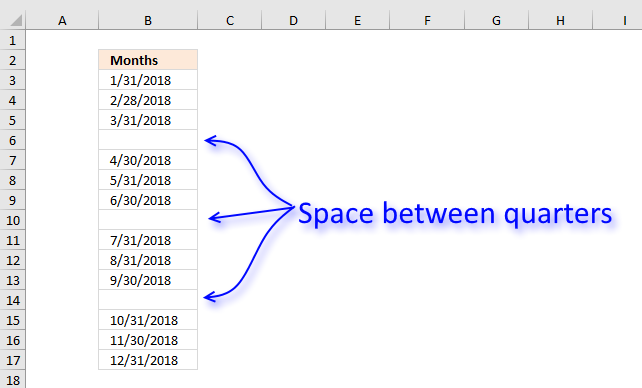Author: Oscar Cronquist Article last updated on December 01, 2018Question: How do I create a list of dates with blanks between each quarter? (Q1, Q2, Q3 and Q4)

Formula in B3:

=IF(MOD(ROWS(\$A\$1:A1), 4), TEXT(DATE(2018, SUMPRODUCT((\$B\$2:B2<>"")*1)+1, 1)-1, "M/D/YYYY"), "")

If you don't need the formula to be dynamic, like in a dashboard or an interactive sheet then simply follow Jarek's instructions below.

1. Create a list of 3 months in a quarter
2. Select it together with a blank cell beneath
3. Drag down

Thanks!

### Explaining formula in cell B3

#### Step 1 - Check blank cells above

We want to know how many cells above have been populated with dates in order to calculate the next date.

\$B\$2:B2<>""

becomes

"Months"<> ""

and returns FALSE.

#### Step 2 - Sum values

The SUMPRODUCT function can't sum boolean values, we need to convert them into their numerical equivalents.

SUMPRODUCT((\$B\$2:B2<>"")*1)+1

becomes

SUMPRODUCT((FALSE)*1)+1

becomes

SUMPRODUCT(0)+1

becomes

0+1 and returns 1.

#### Step 3 - Create date

The DATE function creates an Excel date based on year, month and day.

DATE(2018, SUMPRODUCT((\$B\$2:B2<>"")*1)+1, 1)

becomes

DATE(2018, 1, 1)

and returns 43132.

#### Step 4 - Convert the Excel date to something we understand

The TEXT function can do many things, one of them is to format an Excel date.

TEXT(DATE(2018, SUMPRODUCT((\$B\$2:B2<>"")*1)+1, 1)-1, "M/D/YYYY")

becomes

TEXT(43132, "M/D/YYYY")

and returns 1/31/2018 in cell B3.

#### Step 5 - Create a blank between quarters

The IF function uses the logical expression to determine if a date or blank is to be returned.

IF(MOD(ROWS(\$A\$1:A1), 4), TEXT(DATE(2018, SUMPRODUCT((\$B\$2:B2<>"")*1)+1, 1)-1, "M/D/YYYY"), "")

becomes

IF(MOD(ROWS(\$A\$1:A1), 4), "1/31/2018", "")

The MOD function returns the remainder after a number is divided, this allows you to create a squency of values that repeats 1, 2, 3, 0, 1, 2,3, ... and so on.

IF(MOD(ROW(A1), 4), "1/31/2018", "")

becomes

IF(1, "1/31/2018", "")

and returns "1/31/2018" in cell B3.